# # 《程序员数学：位运算》—— 如何使用二进制计算乘法？

## # 一、前言

`你是什么时候注意到位运算？`

``````int a = 2, b = 3;
a = a ^ b;
b = a ^ b;
a = a ^ b;
``````
1
2
3
4

^ 异或运算：两个操作数的同位中，如果值相同（都是 0 或者都是 1）则为 0，不同（一个是 0，一个是 1）则为 1

• 以二进制数据为基础进行运算解析
• a = 2 二进制数为 0010、b = 3 二进制数为 0011
• a = a ^ b = 0010 ^ 0011 = 0001
• b = a ^ b = 0001 ^ 0011 = 0010 = 2
• a = a ^ b = 0001 ^ 0010 = 0011 = 3
• 异或运算的基本定理解析
• a = a ^ b
• b = a ^ b = a ^ b ^ b = a = 2
• a = a ^ b = a ^ a ^ b = b = 3

## # 二、位操作介绍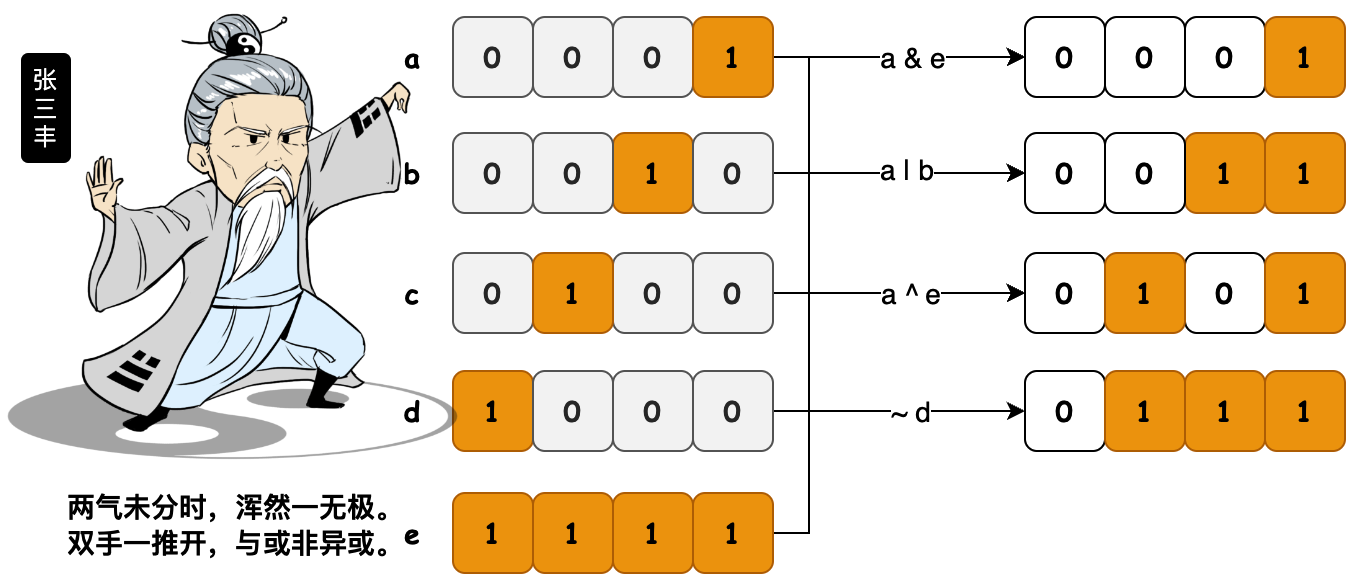``````int a = 1; // 0001
int b = 2; // 0010
int c = 4; // 0100
int d = 8; // 1000
int e = 15;// 1111

// 与运算；0001
System.out.println(Integer.toBinaryString(a & e)); // 0001
// 或运算；0011
System.out.println(Integer.toBinaryString(a | b)); // 0011
// 异或运算；0101
System.out.println(Integer.toBinaryString(a ^ c)); // 0101
// 非运算；...11110111
System.out.println(Integer.toBinaryString(~d));
``````
1
2
3
4
5
6
7
8
9
10
11
12
13
14
• 与运算；两个数都转为二进制，然后从高位开始比较，如果两个数都为1则为1，否则为0。
• 或运算；两个数都转为二进制，然后从高位开始比较，两个数只要有一个为1则为1，否则就为0。
• 非运算；如果位为0，结果是1，如果位为1，结果是0。
• 异或运算；两个数转为二进制，然后从高位开始比较，如果相同则为0，不相同则为1。

## # 三、位运算案例

### # 1. 获取位值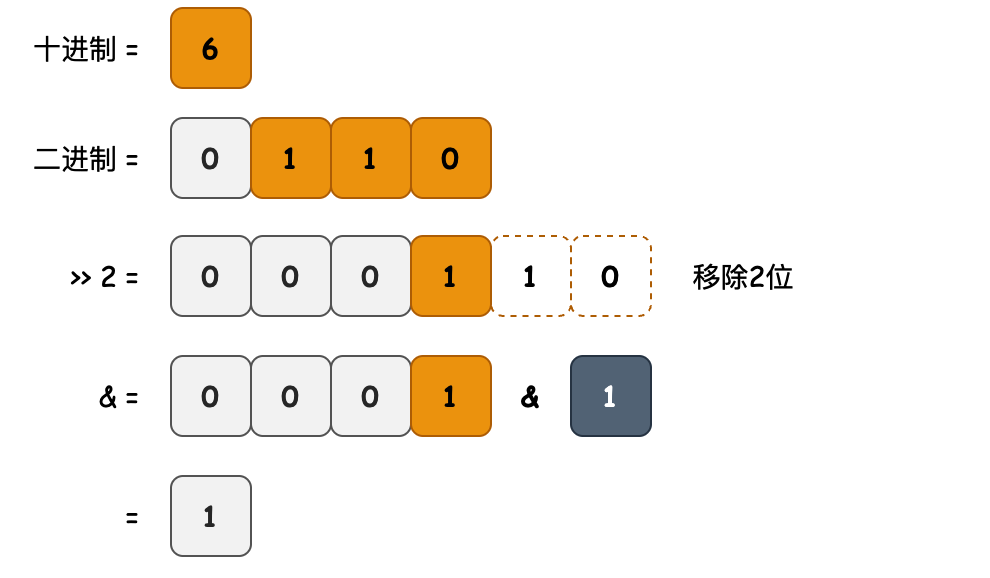``````public int getBit(int number, int bitPosition) {
return (number >> bitPosition) & 1;
}
``````
1
2
3
• 目的：获取二进制数字中，指定位置的值。
• 逻辑：该方法将目标值右移到最右边，即位数组的第0个位置上，如；0001 的二进制形式。之后与 1 进行与操作。如果目标位是1，那么结果就是1，反之结果是0；

### # 2. 设置位值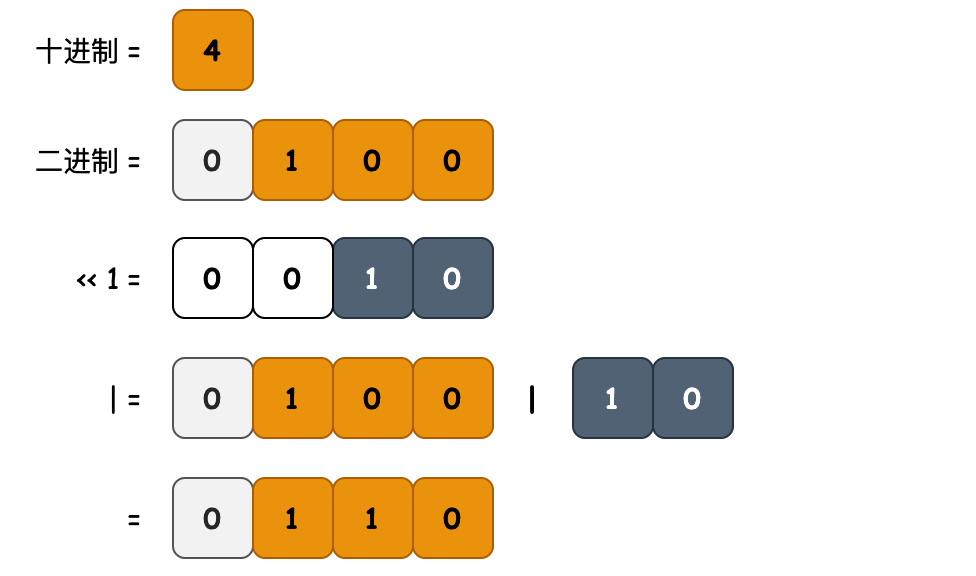``````public int setBit(int number, int bitPosition) {
return number | (1 << bitPosition);
}
``````
1
2
3
• 目的：设置二进制数字中，指定位置的值
• 逻辑：1 就像一个子弹，左移指定位数到目标位置，如；0010 的二进制形式。与目标值 number 做或运算(把子弹打进去)，设置结果并返回。

### # 3. 清空位值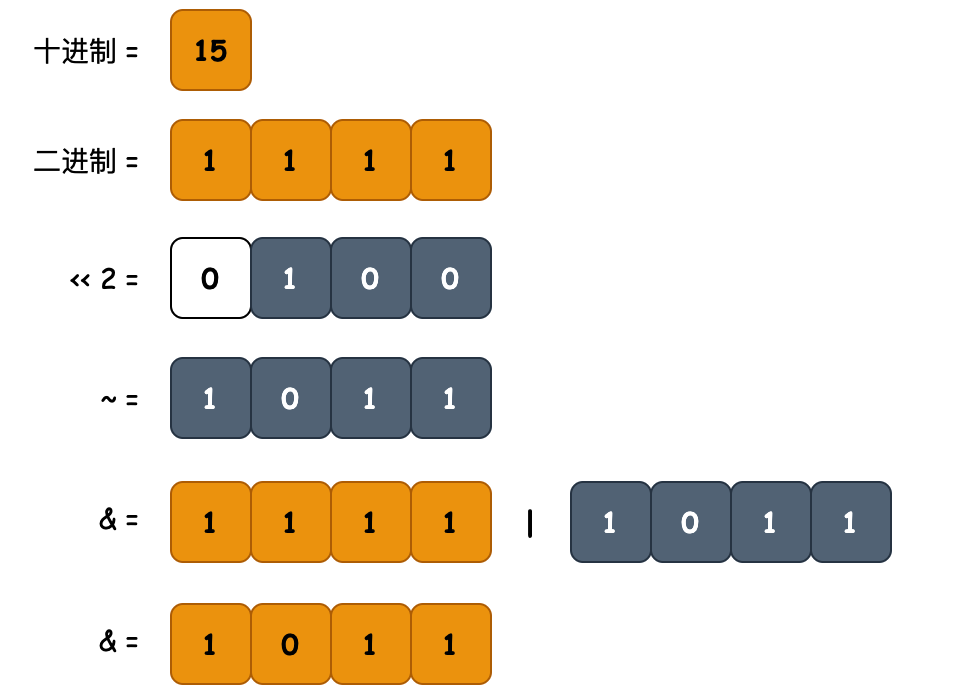``````public int clearBit(int number, int bitPosition) {
int mask = ~(1 << bitPosition);
}
``````
1
2
3
4
• 目的：清空二进制数字中，指定位置的值
• 逻辑：类似于`设置位值`，把1左移指定位数后取反，从 0010 得到 1101 并与目标值 number 做与&运算，清掉目标位的值。

### # 4. 更新位值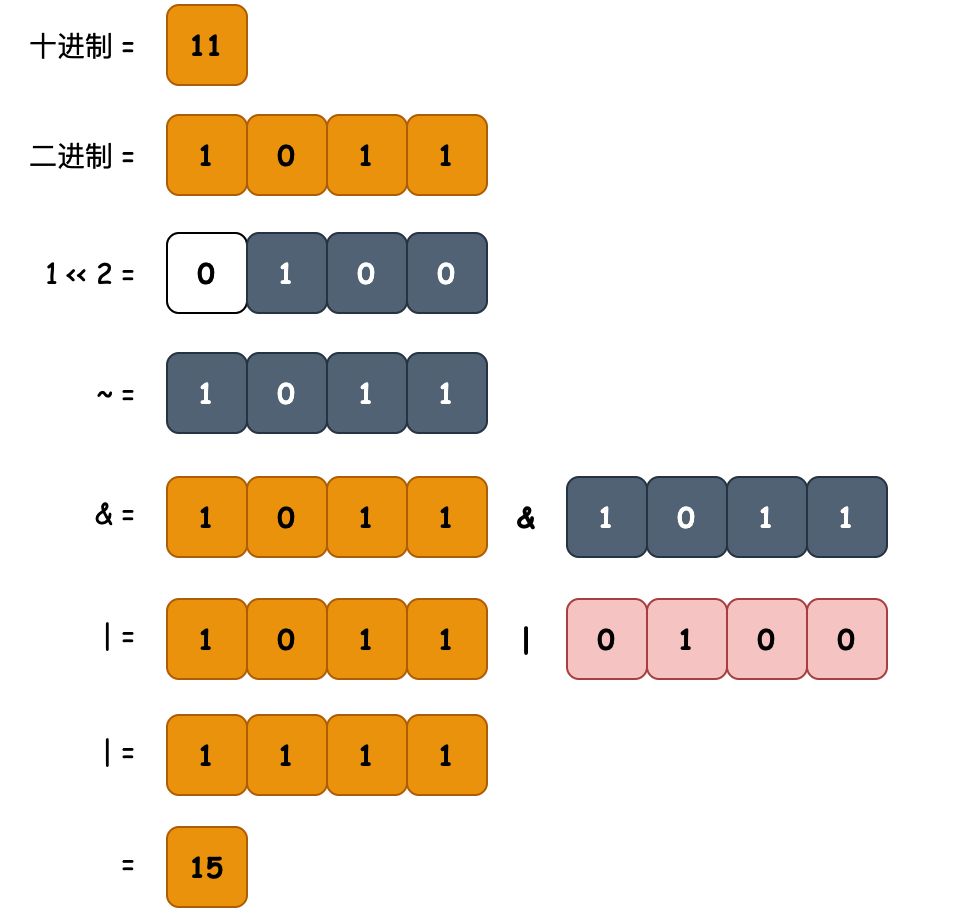``````public int updateBit(int number, int bitPosition, int bitValue) {
int clearMask = ~(1 << bitPosition);
return (number & clearMask) | (bitValue << bitPosition);
}
``````
1
2
3
4
• 目的：清空二进制数字中，指定位置的值
• 逻辑：结合清空clearBit、设置setBit，两个方法将制定位置替换为设置值。

### # 5. 偶数判断``````public boolean isEven(int number) {
return (number & 1) == 0;
}
``````
1
2
3
• 目的：检测 number 是否为偶数
• 逻辑：检测二进制的最右侧一位，如果是1，那么一定是奇数。所以可以与1做与&运算的结果和0判断。不等于0是奇数，等于0是偶数。

### # 6. 正数判断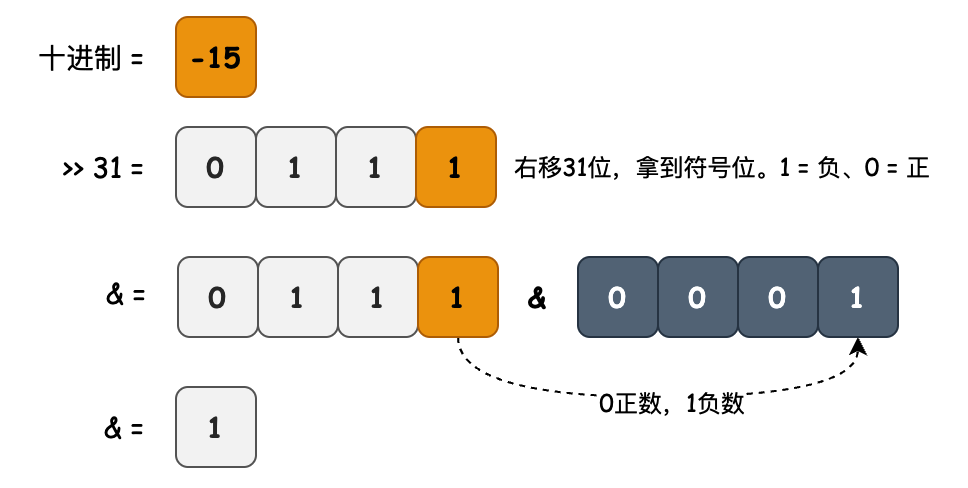``````public boolean isPositive(int number) {
if (number == 0) {
return false;
}
return ((number >> 31) & 1) == 0;
}
``````
1
2
3
4
5
6
• 目的：判断 number 值是否为正数。
• 逻辑：基于二进制正数最左边的值是0的这个事实，右移31位，和1做与&运算，如果结果等于1为负数，反正为正数。

### # 7. 左移乘二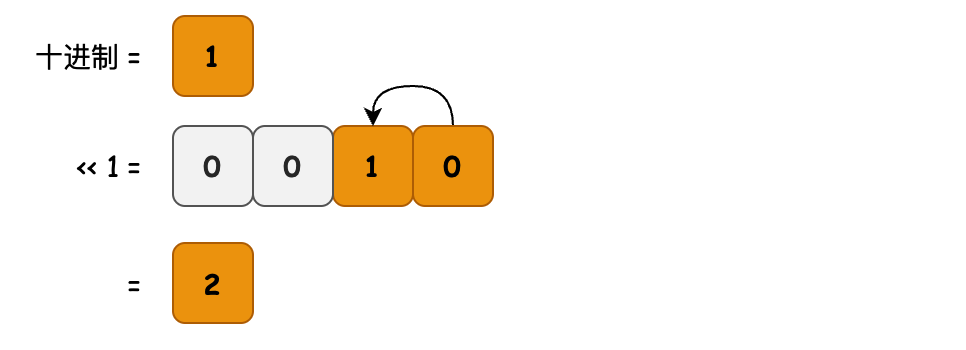``````public int multiplyByTwo(int number) {
return number << 1;
}
``````
1
2
3
• 目的：乘以2
• 逻辑：该方法将原始数字向左移动一位。因此所有位都将乘以2，因此数字本身也将乘以2。

### # 8. 右移除二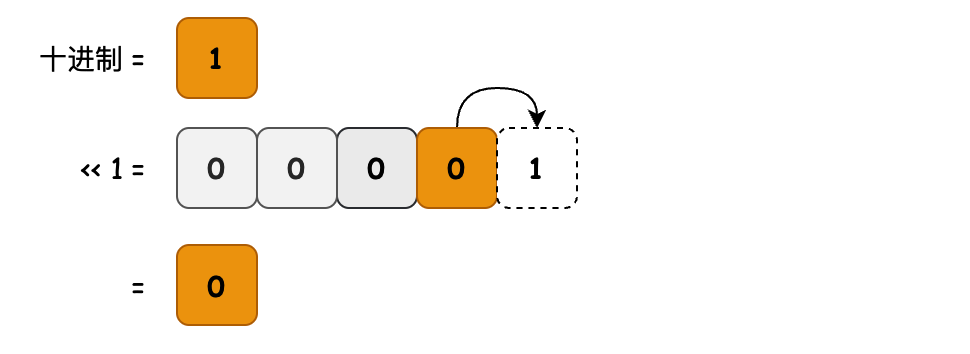``````public int divideByTwo(int number) {
return number >> 1;
}
``````
1
2
3
• 目的：除以2
• 逻辑：该方法将原始数字向右移动一位。因此所有位都将除以2，因此数字本身也将除以2,且不会产生余数。

### # 9. 正负交换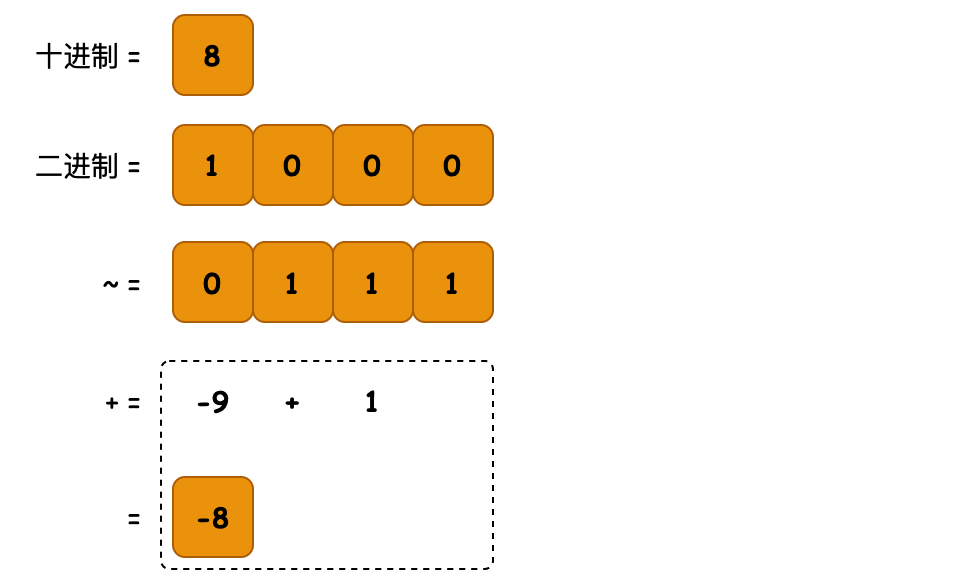``````public int switchSign(int number) {
return ~number + 1;
}
``````
1
2
3
• 目的：正数转负数，负数转正数
• 逻辑：通过二进制取反运算，如 1000 = 8 取反 1.....0111 = -9 + 1 = -8

### # 10. 乘法运算(有符号)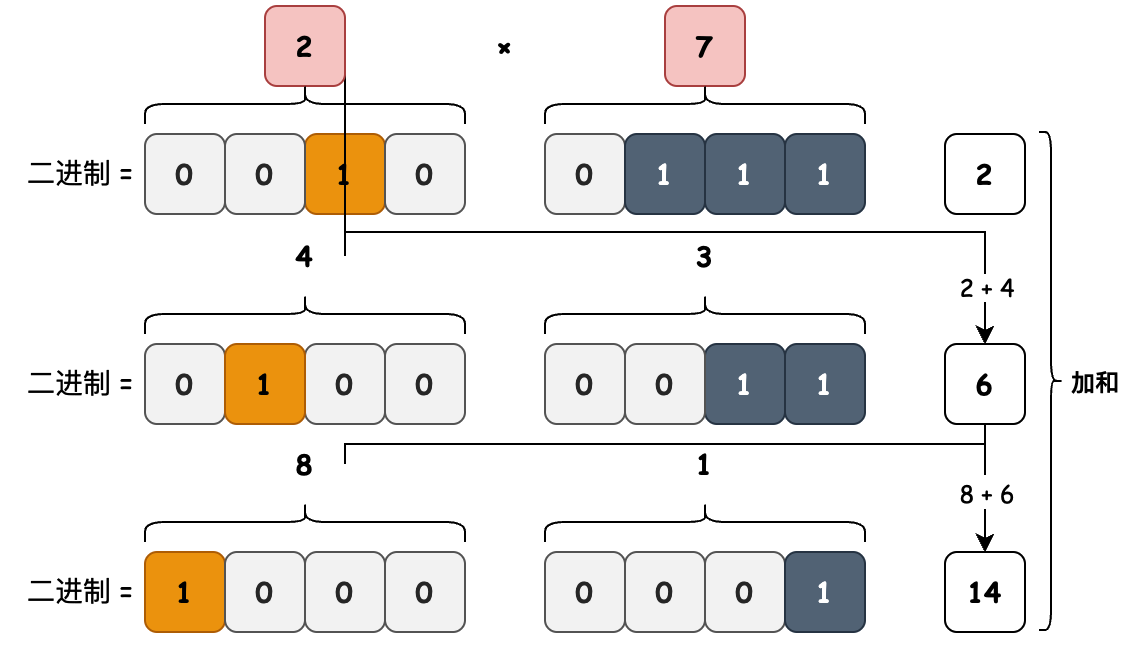``````public int multiply(int a, int b) {
int multiply = 0;
while (a != 0 && b != 0) {
System.out.print("计算步骤(" + (isEven(b) ? "偶数" : "奇数") + ")：a(" + String.format("%04d", Integer.valueOf(Integer.toBinaryString(a))) + ") = " + a + " | b(" + String.format("%04d", Integer.valueOf(Integer.toBinaryString(b))) + ") = " + b);
// b 是偶数：2a * (b/2)
if (isEven(b)) {
a = multiplyByTwo(a);
b = divideByTwo(b);
}
// b 奇数
else {
// b 正数：2a * (b - 1)/2 + a
if (isPositive(b)) {
multiply += a;
a = multiplyByTwo(a);
b = divideByTwo(b - 1);
}
// b 负数：2a * (b + 1)/2 - a
else {
multiply -= a;
a = multiplyByTwo(a);
b = divideByTwo(b + 1);
}
}
System.out.println(" | multiply(" + String.format("%04d", Integer.valueOf(Integer.toBinaryString(multiply))) + ") = " + multiply);
}
return multiply;
}
``````
1
2
3
4
5
6
7
8
9
10
11
12
13
14
15
16
17
18
19
20
21
22
23
24
25
26
27
28
• 目的：计算有符号二进制乘积
• 公式：推到公式与代码向对应
• = a * b
• = 2a * (b/2) —— b为偶数
• = 2a * (b - 1)/2 + a —— b 为奇数、正数
• = 2a * (b + 1)/2 - a —— b 为奇数、负数
• 逻辑：乘数a不断左移、乘数b不断右移。当b归0时，a左移累计下来的值就是乘积总和。如图

### # 11. 乘法运算(无符号)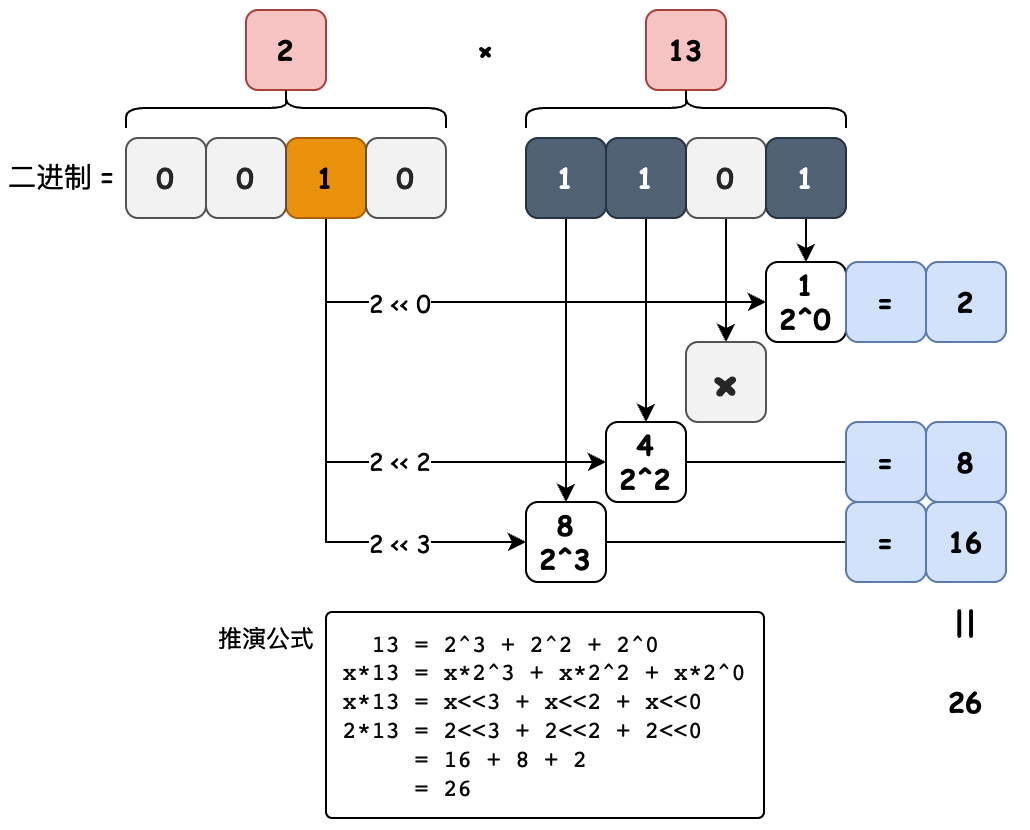``````public int multiplyUnsigned(int number1, int number2) {
int result = 0;
int multiplier = number2;
int bitIdx = 0;
while (multiplier != 0) {
if ((multiplier & 1) == 1) {
System.out.println(number1 + " << " + bitIdx + " = " + (number1 << bitIdx));
result += number1 << bitIdx;
}
bitIdx += 1;
multiplier = multiplier >> 1;
}
return result;
}
``````
1
2
3
4
5
6
7
8
9
10
11
12
13
14
• 目的：计算无符号二进制乘积

• 公式

• `13 = 2^3 + 2^2 + 2^0`
• `x*13 = x*2^3 + x*2^2 + x*2^0`
• `x*13 = x<<3 + x<<2 + x<<0`
• `2*13 = 2<<3 + 2<<2 + 2<<0`
• `= 16 + 8 + 2`
• `= 26`
• 逻辑：每个数字都可以表示成一系列2的幂之和。例如 13 的二进制是 1101，最右侧第1位1，是2的0次幂，所以对应2的进制值是左移0位。再比如13的右数第3位是1，对应位置值是4也就是2的2次幂，所以对应2的进制值是左移2位。最终把这些值相加就是乘积值。

### # 12. 一的数量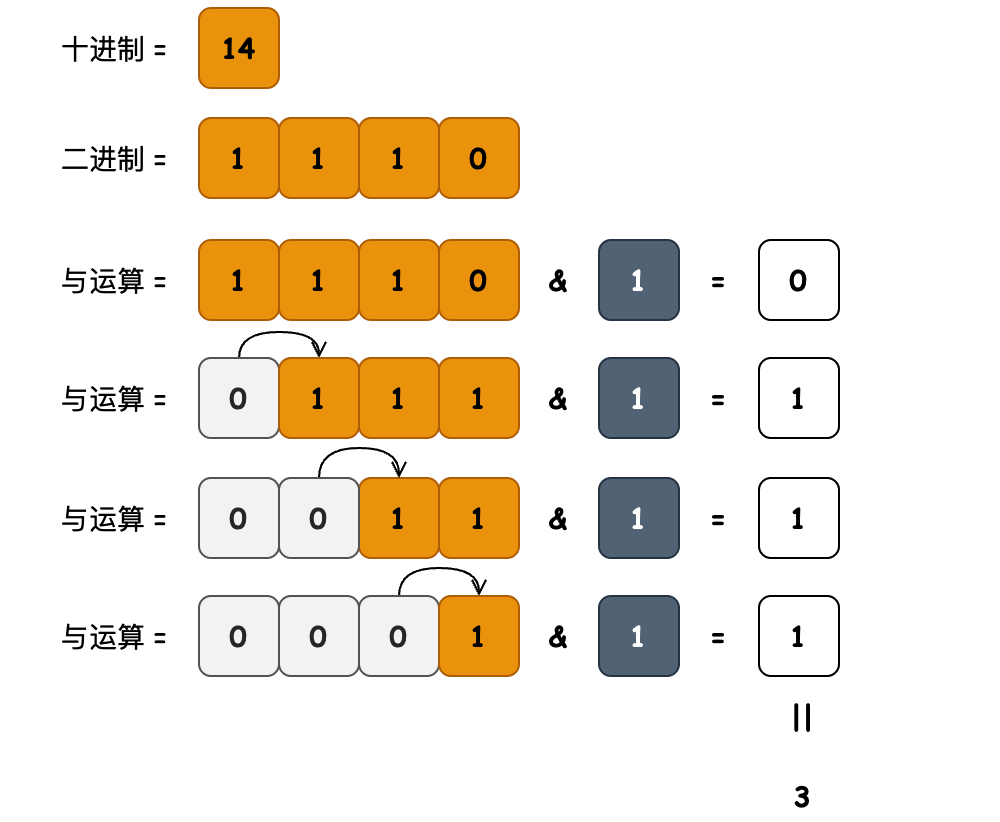``````public int countSetBits(int originalNumber) {
int setBitsCount = 0;
int number = originalNumber;
while (number != 0) {
setBitsCount += number & 1;
number >>>= 1;
}
return setBitsCount;
}
``````
1
2
3
4
5
6
7
8
9
• 目的：使用位运算符对一个数字里设置为1的位进行记数
• 逻辑：把数字每次向右移动1位，然后使用&操作符取出最右边一位的值，1则记数加1，0则不计。

### # 13. 转换计算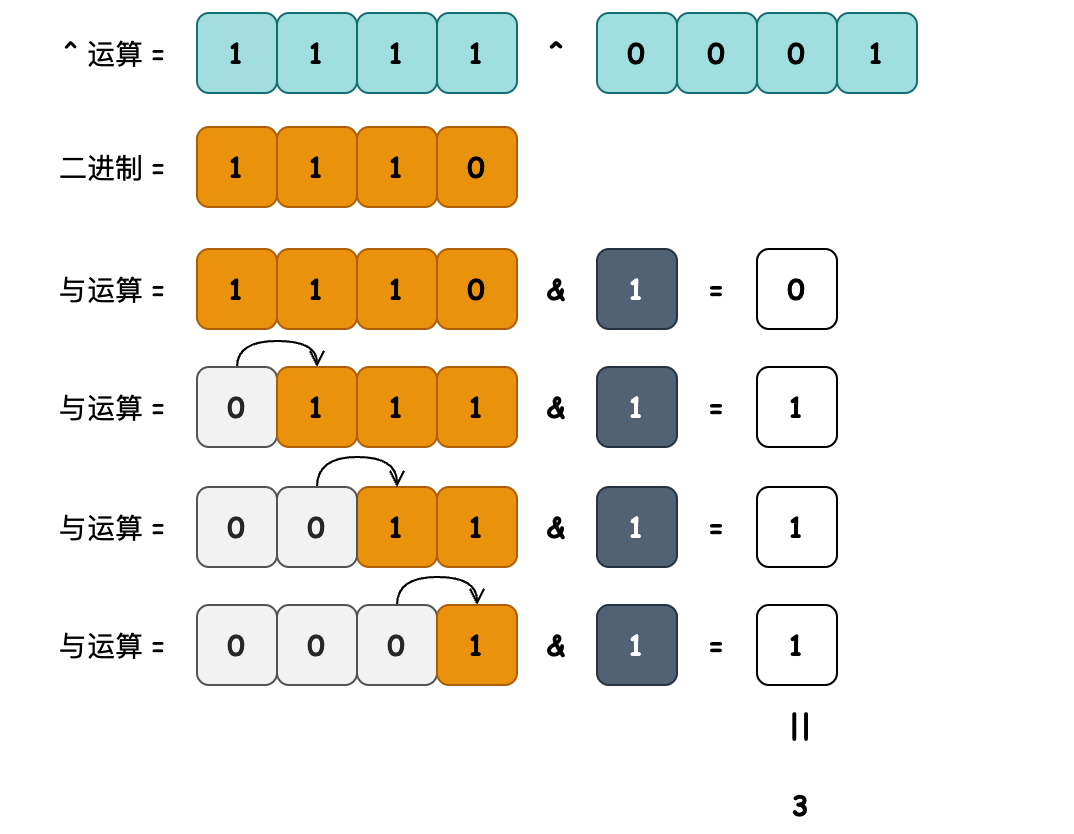``````public int bitsDiff(int number1, int number2) {
return countSetBits(number1 ^ number2);
}
``````
1
2
3
• 目的：计算一个数字，转换为另外一个数字，所需要的转换位数。
• 逻辑：当数字进行XOR异或运算时，结果将是不同位数的数量(即异或的结果中所有被设置为1的位的数量)。

### # 14. 有效位数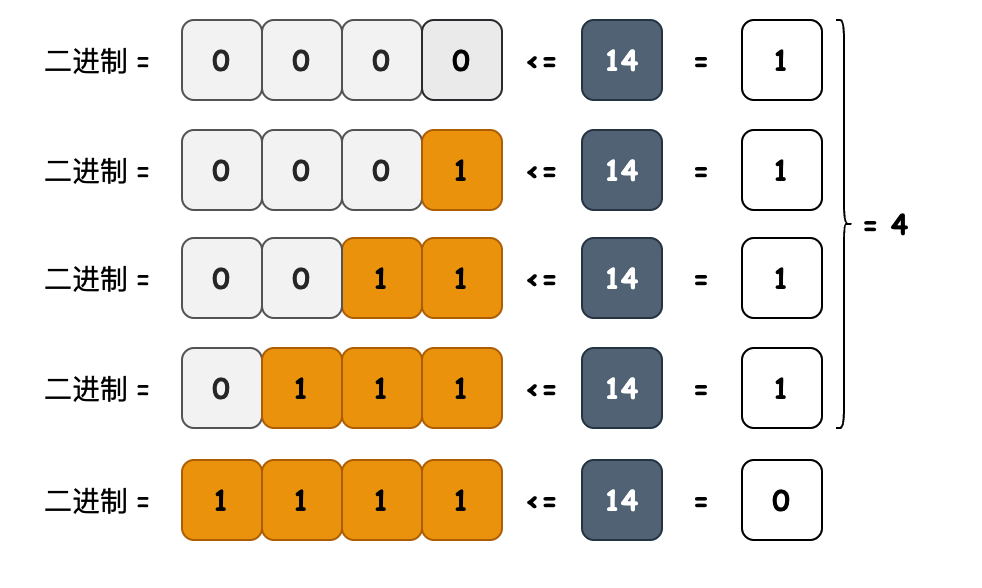``````public int bitLength(int number) {
int bitsCounter = 0;
while ((1 << bitsCounter) <= number) {
bitsCounter += 1;
}
return bitsCounter;
}
``````
1
2
3
4
5
6
7
• 目的：计算二进制数值的有效位数，例如 14 = 1110 有效位为4位。
• 逻辑：通过1不断地左移加和与 number 做对比，只要比number小就累加1位。

### # 15. 幂值判断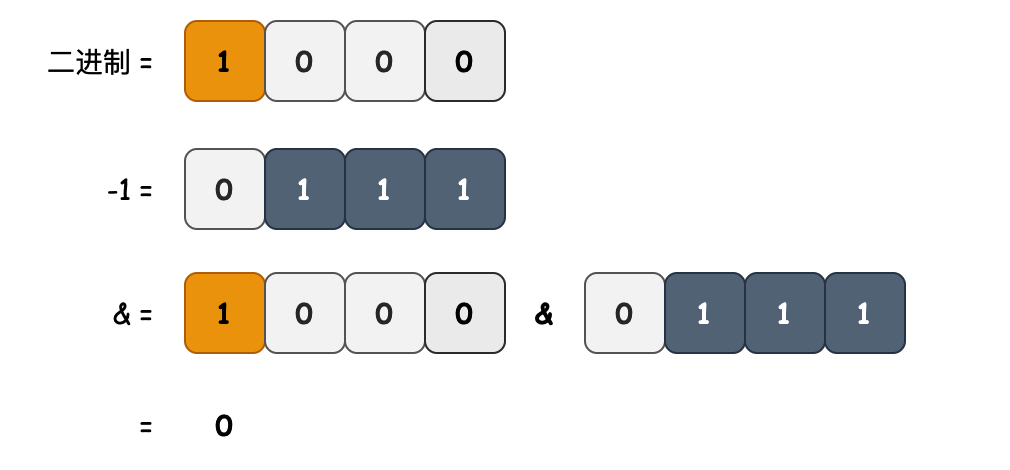``````public boolean isPowerOfTwo(int number) {
return (number & (number - 1)) == 0;
}
``````
1
2
3
• 目的：检查number是否为2的幂值。
• 逻辑：2的幂值形式的数字为2、4、8、16 等，那么可以把一个二进制数进行错位与&运算，如果错位比对都为0，那么就是2的幂数。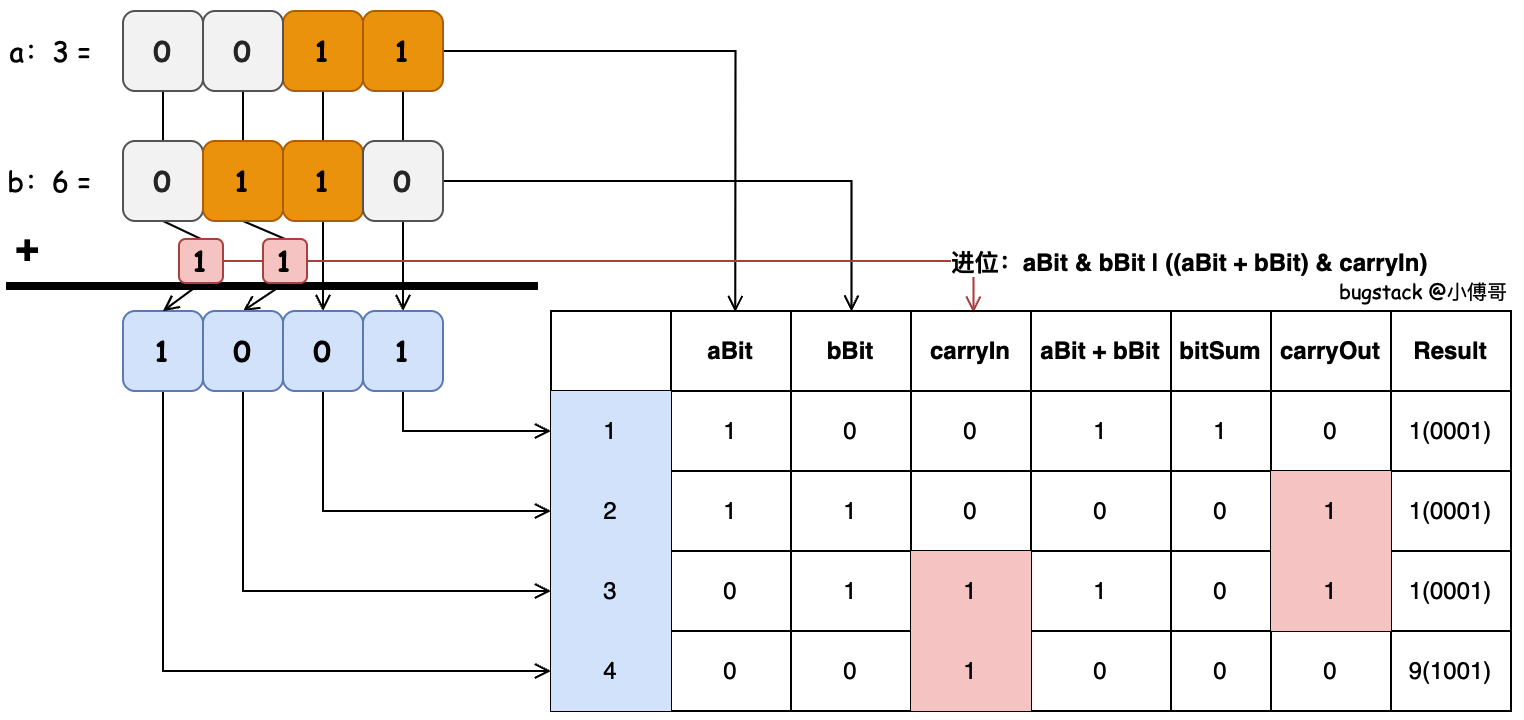``````public int fullAdder(int a, int b) {
int result = 0;
// 计算每次的进位值，1 + 1 = 0010 进位为1。是一种&运算。
int carryOut = 0;
System.out.println("| aBit | bBit | carryIn | aiPlusBi | bitSum | carryOut | result |");
for (int i = 0; i < 32; i++) {
int aBit = getBit(a, i);
int bBit = getBit(b, i);
int carryIn = carryOut;
System.out.print("|   " + aBit + "  |  " + bBit + "   |       " + carryIn);
// 加和 - 两个值；如果相同则为0，不相同则为1
int aiPlusBi = aBit ^ bBit;
System.out.print(" |        " + aiPlusBi);

// 加和 - 进位；
int bitSum = aiPlusBi ^ carryIn;
System.out.print(" |      " + bitSum);

// 进位；同位置 ai & bi = 1 | 与进位 aiPlusBi & carryIn = 1
carryOut = (aBit & bBit) | (aiPlusBi & carryIn);
System.out.print(" |  " + carryOut + "(" + Integer.toBinaryString(carryOut) + ")   ");

// 累加；把当前位置计算的值，左移n位
result = result | (bitSum << i);
System.out.println(" | " + result + "(" + String.format("%04d", Integer.valueOf(Integer.toBinaryString(result))) + ")|");
}
return result;
}
``````
1
2
3
4
5
6
7
8
9
10
11
12
13
14
15
16
17
18
19
20
21
22
23
24
25
26
27
28
• 目的：计算有符号二进制加法
• 逻辑：二进制的累加可以对照下计算10进制累加时一样，对应2个数字相加，当有进位的时候记录进位。
• 首先二进制的加和计算，1+1 = 10、1+0=01、0+1=01、0+0=00，那么正好对应上 ^ 非运算，相同则为0，不相同则为1，因为即使两个1相加，当前位的值也是0。
• 之后是进位相加，两数想加后，还可能有进位上来的数值与两数进行相加。
• 结果相加完成后，计算进位，并保留进位用于下次计算。进位的计算为；ai & bi = 1 | 与进位 aiPlusBi & carryIn = 1，无论是两数相加，还是两数的和 aiPlusBi 与进位相加，只要与运算是1，那么就要保留进位。
• 最后是累加结果，把对应位置的结果计算，按照当前计算到到二进制的位数左移到目标为止，累加到 result，最后就是结果值。

## # 四、常见面试题

• & 和 ~ 是什么运算？
• 两数交换不引入第三个变量如何处理？
• 二进制中1个个数怎么计算？
• 实现一个两数加和？
• 实现一个无符号两数成绩？